## 4) Number Before

Counting: Lesser Number

### Mathematics

• Develop fluency identifying the number before, for numbers up to ten.

Representing the number after on a number track.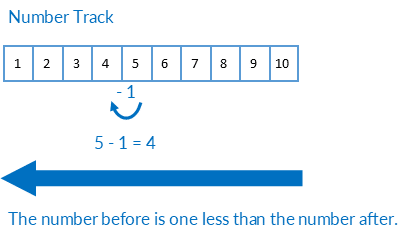For example, the number after five.Representing the number before on a number line.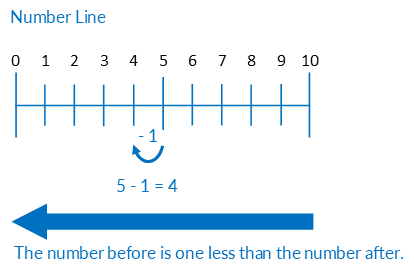For example, the number before five.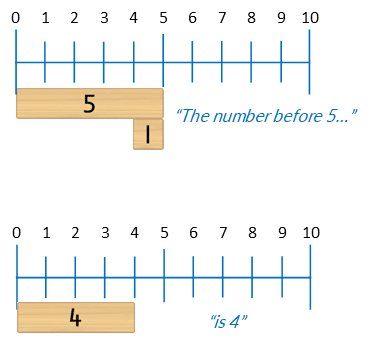### Language

• number before
• one less than
• one fewer than

When initially completing the activity introduce and use the language “the number after”. In subsequent sessions, once students are fluent with the activity, change the language to “one greater than” and later still “one more than”.

### Mental Number Lines

One use for number lines is counting forwards and backwards. For example, forwards from zero or backwards from ten.  Please note, this is not how the number lines are to be used in these activities.

In these activities number lines are used to develop the concept of a mental number line, that is, where numbers are located in relation to each other. If students need to count to identify the location of a number they should only count on/back one, two or three. Counting more than this is inefficient, error prone and limits opportunities for students to develop more effective strategies.

• Activity 2 focuses on locating the number after.
• Activity 4 focuses on locating the number before.
• As students progress through the Bond Blocks system they will develop the skills needed to locate harder to identify numbers such as 7 and 8.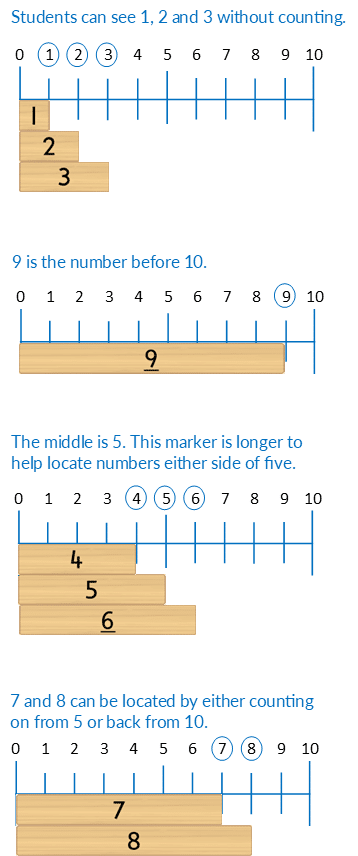## Differentiation

### A little easier

##### Alternating numbers

Remove every second block from the steps. Build flat on a desk. Students count aloud, pointing to each number as it is said.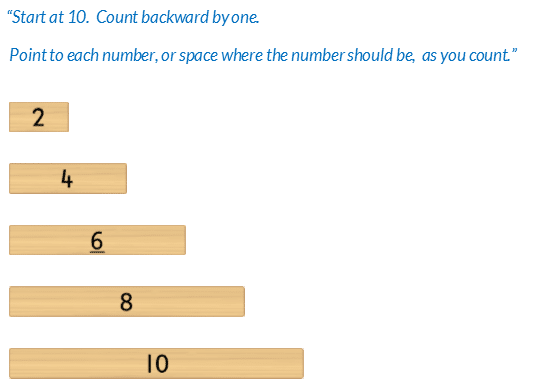Next, repeat this activity but the student takes turns saying the counting numbers with the teacher. The teacher points to and says the number that is represented with Bond Blocks. The student points to the space before this block and says the number that is missing.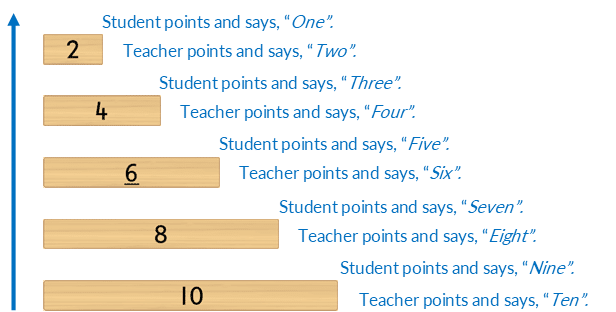Then, repeat counting with alternating numbers, taking turns with the teacher, but introduce the language, “The number before”. The teacher points to the Bond Block that is placed and says, “The number before ten is…” The student points to the space after Block Ten and says “Nine.”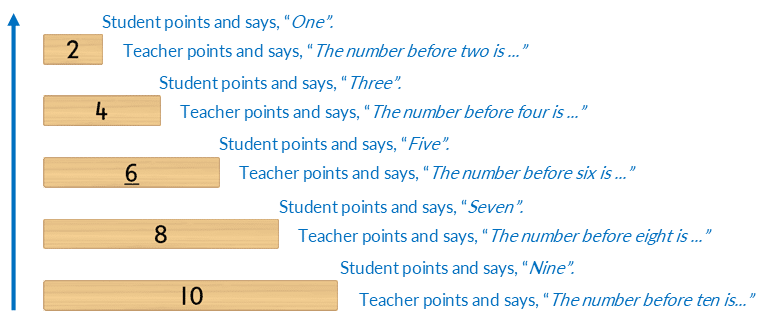Once students are fluent using the alternating steps made with even numbers repeat using the odd numbered Bond Blocks.

### A little harder

##### Counting number before

Complete the ‘a little harder’ activity board.

##### Even steps

Count backwards, subtracting two each time, starting at 10, with blocks, to 2.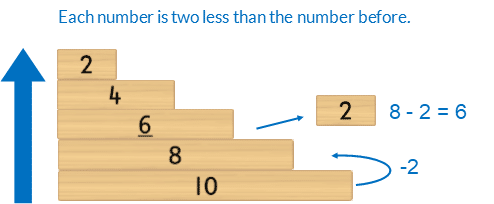Extend to counting backwards with even numbers, by two, from 18.Count backwards, subtracting two each time, starting at 10, without blocks, to 2. Extend to counting backwards by two, without blocks, from 18.

##### Odd steps

Count backwards, subtracting two each time, starting at 9, with blocks, to 1.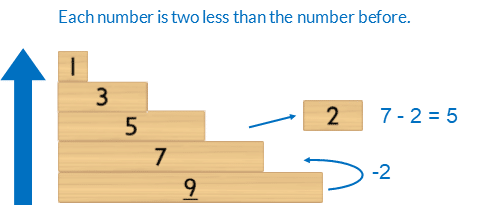Extend to counting backwards with odd numbers, by two, from 19.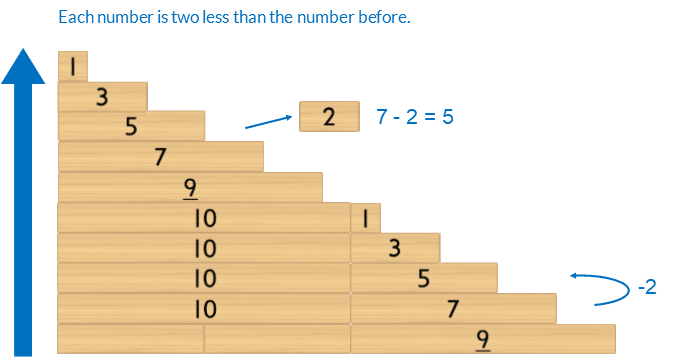Count backwards, subtracting two each time, starting at 9, without blocks, to 1. Extend to counting backwards by two, without blocks, from 19.

##### Fluency

Develop fluency counting backwards when packing away. Cover the template with a piece of white paper that is 22cm x 20cm.

Give students a different instruction each time they pack away. For example:

• Pack away the even blocks first.
• Pack away the odd blocks first.
• Pack away the even blocks first, starting at 10, counting backwards.
• Pack away the odd blocks first, starting at 9, counting backwards.

### Progression

In the next activity students identify individual numbers in the counting sequence to 10 without counting. Go to

##### Activity 5

Counting: Identifying Numbers 6 to 10, Building Steps